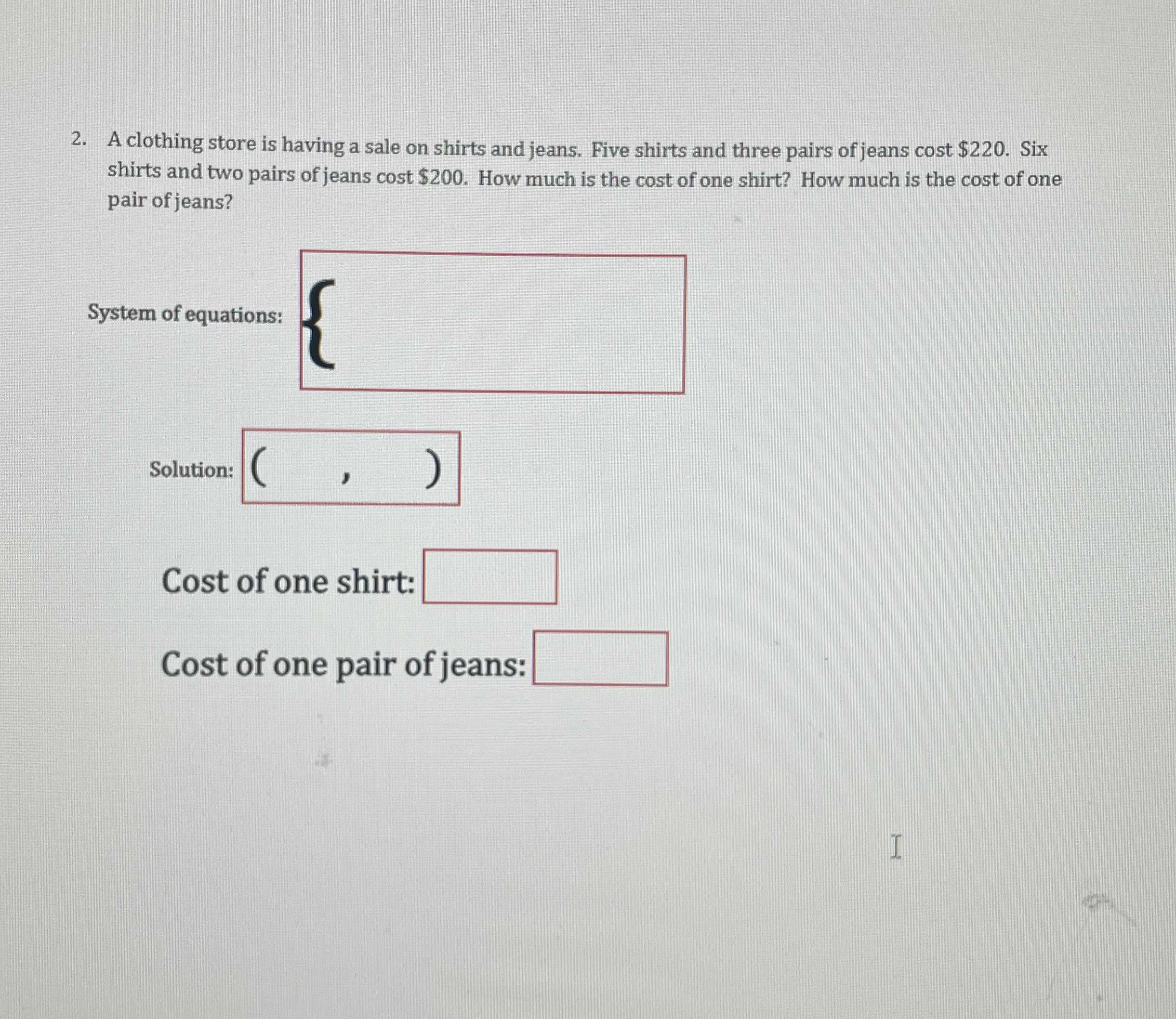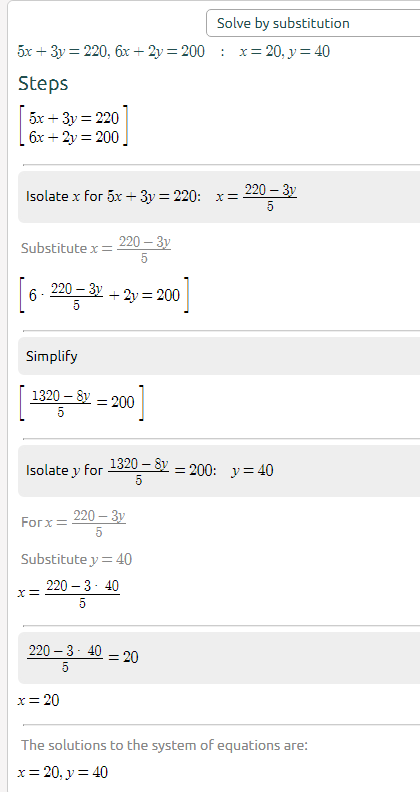### ¿Todavía tienes preguntas de matemáticas?

Pregunte a nuestros tutores expertos
Algebra
PreguntaA clothing store is having a sale on shirts and jeans. Five shirts and three pairs of jeans cost $$\ 220$$ . Six shirts and two pairs of jeans cost $$\ 200$$ . How much is the cost of one shirt? How much is the cost of one pair of jeans?

System of equations:

Solution:

Cost of one shirt:

Cost of one pair of jeans:Cost of 1 shirt is $20 Cost of 1 pair of jeans is$40